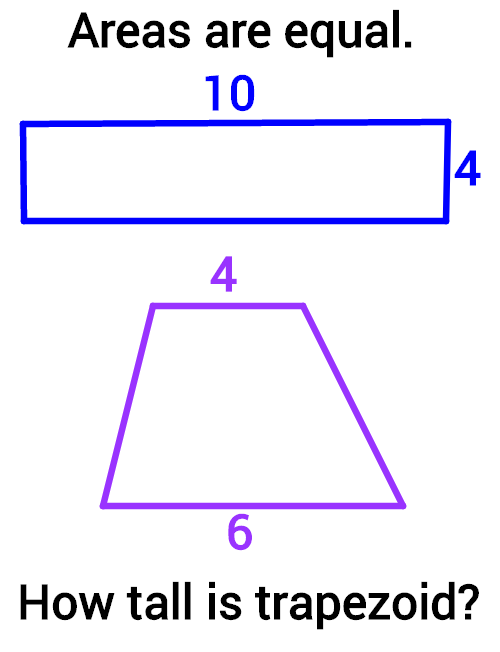Geometry

# Calculating Areas

## Intro

Understanding and applying area concepts allows us create robots, airplanes, stadiums, pools, houses, and more.

Expect to see and learn how to solve questions like this one:Area describes how much two-dimensional space something occupies, or how many unit squares fit into a given figure. Area helps us determine how much much paint, or carpet, or soil we need. From areas of lawns and streets to dimensions of i-phones and airplane wings, the applications of area are limitless and used in nearly every aspect of everyday life.

Examining areas of different shapes helps us understand how common mathematical formulas are created and how various shapes relate to one another. Our study of area extends the foundational knowledge of rectangular areas to develop a deep understanding of areas of triangles, parallelograms, trapezoids, and circles.

Area concepts set the essential foundation for examining other geometric properties such as volume and surface area.

×